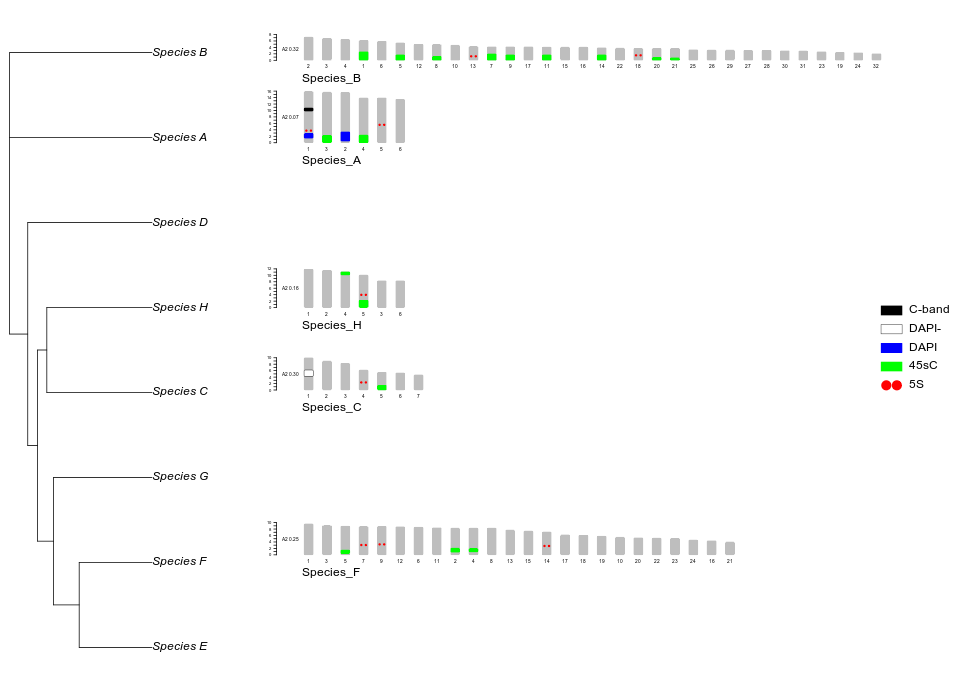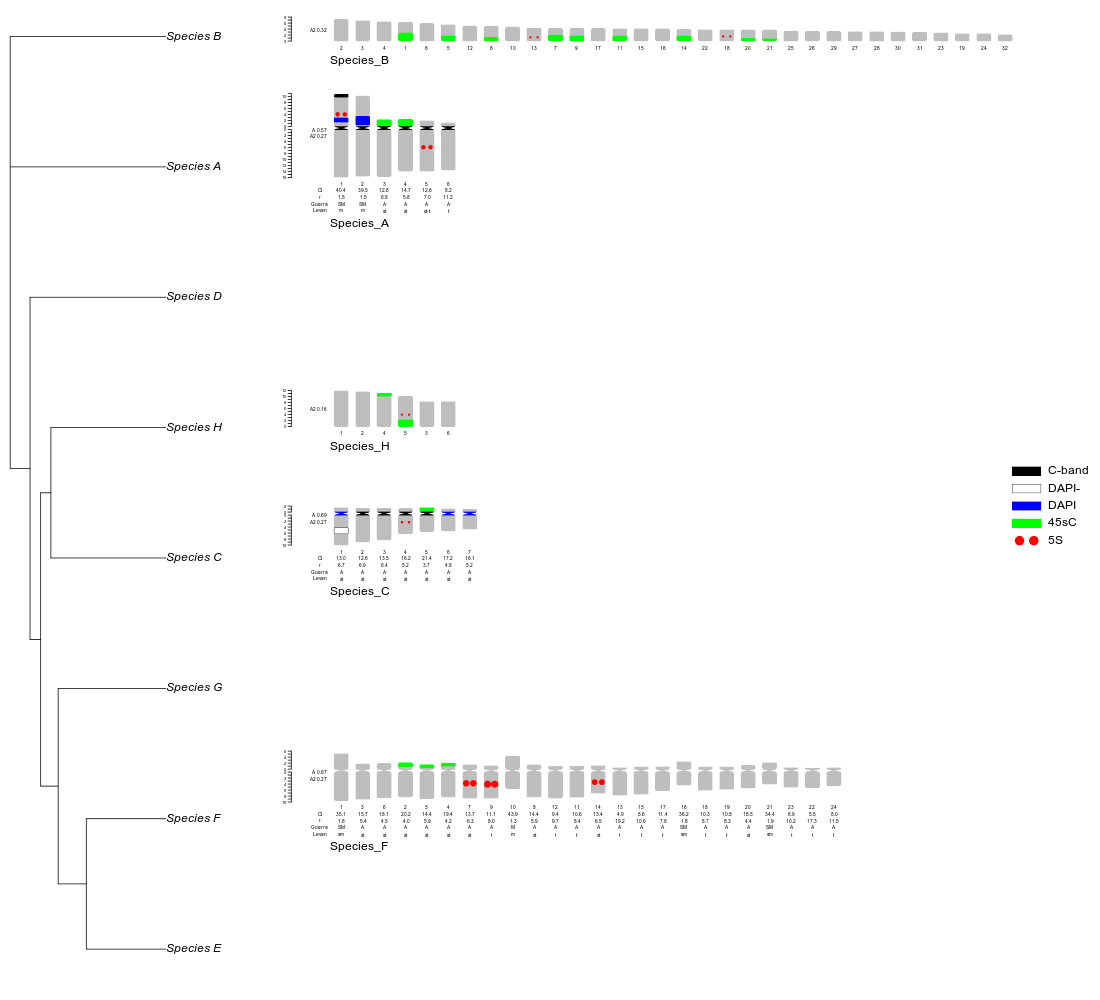This guide shows the files to plot idiograms alongside a phylogeny

visit gitlab for installation instructions https://gitlab.com/ferroao/idiogramFISH

#load package
library(idiogramFISH) 

# 2 ggtree of iqtree and monocentrics

idiogramFISH comes with two trees and data.frames with chr. and marks’ data for the correspondent OTUs, first we will plot a tree produced with iqtree (Nguyen et al., 2015)

We will use phytools for that (Revell, 2012)

require(ggplot2)
require(phytools)
require(ggpubr)
require(grid)   #pushViewport
require(ggtree)
# list.files(system.file('extdata', package = 'my_package') )

# find path of iqtree file
iqtreeFile    <- system.file("extdata", "eightSpIqtree.treefile", package = "idiogramFISH")

# load file as phylo object

# transform tree
iqtreephyloUM <- force.ultrametric(iqtreephylo, method= "extend") # phytools

## Make a ggtree (Yu et al., 2018)

ggtreeOf8 <- ggtree(iqtreephyloUM) + geom_tiplab(size=6)

Modify optionally graphical parameters with ggplot and ggpubr: (Wickham, 2016; Kassambara, 2019)

gbuil2      <-  ggplot_build(ggtreeOf8)       # get ggplot_built
gtgbuild    <-  ggplot_gtable(gbuil2)         # get gtable from ggplot_built
gtgbuild$layout$clip[gtgbuild$layout$name == "panel"] <- "off"                # modify gtable
ggtreeOf8b   <- as_ggplot(gtgbuild)            # back to ggplot
gtgbuildgg2 <- ggtreeOf8b +  theme(plot.margin = unit(c(1,9.5,3,1.5), "cm") ) # top right bottom left - modify margins

Our plot of the tree is ready, let’s get the order of species in tree

ggtreeOf8TIPS<-ggtreeOf8$data[which(ggtreeOf8$data$isTip),] desiredOrder <- rev(ggtreeOf8TIPS[order(ggtreeOf8TIPS$y),]$label) ## Order OTUs of data.frame of chr. data Apply order of phylogeny to data.frame # make a vector without missing OTUs desiredFiltered <- intersect(desiredOrder,allChrSizeSample$OTU)

# establish desired order
allChrSizeSample$OTU <- factor(allChrSizeSample$OTU, levels = desiredFiltered)

# order
desiredorderRevB <- rev(revBayesPhyloUM$tip.label[ordered_tips]) ## Order OTUs of data.frame of chr. data First, create some data for holocentrics allChrSizeSampleHolo <- allChrSizeSample allChrSizeSampleHolo <- allChrSizeSampleHolo[,c("OTU","chrName","longArmSize")] colnames(allChrSizeSampleHolo)[which(names(allChrSizeSampleHolo)=="longArmSize")]<-"chrSize" allMarksSampleHolo <- allMarksSample allMarksSampleHolo <- allMarksSampleHolo[which(allMarksSampleHolo$chrRegion!="cen"),]
allMarksSampleHolo   <- allMarksSampleHolo[c("OTU","chrName","markName","markDistCen","markSize")]
colnames(allMarksSampleHolo)[which(names(allMarksSampleHolo)=="markDistCen")] <- "markPos"
allMarksSampleHolo[which(allMarksSampleHolo$markName=="5S"),]$markSize <- .5

Apply order of phylogeny to data.frame

# make a vector without missing OTUs
desiredFiltered <- intersect(desiredorderRevB,allChrSizeSampleHolo$OTU) # establish desired order allChrSizeSampleHolo$OTU <- factor(allChrSizeSampleHolo$OTU, levels = desiredFiltered) # order allChrSizeSampleHolo <- allChrSizeSampleHolo[order(allChrSizeSampleHolo$OTU),]

Now we have to establish where are the OTUs in the tree, that don’t have chr. data

# Establish position of OTUs before missing data OTUs
matchres <- match(desiredorderRevB,desiredFiltered)
matchres[is.na(matchres)]   <- "R"
reps     <- rle(matchres)
posOTUsBeforeMissing        <- as.numeric(matchres[which(matchres=="R")-1][which(matchres[which(matchres=="R")-1]!="R")] )

# This are the OTUs that come before missing chr. data OTUs
BeforeMissingPlot2          <- desiredFiltered[posOTUsBeforeMissing]

# This is the amount of missing OTUs, spaces to add (ghost karyotypes)
valuesOfMissRepsBeforePlot2 <- reps$lengths[which(reps$values=="R")]

## Plotting

Now we are ready to plot adding those arguments for addMissingOTUAfter and missOTUspacings

# plot to png file
png(file=paste0("secondplot.png" ),width=962,height=700)

{
par(omi=rep(0,4) , mar=c(0,0,0,0), mfrow=c(1,2))
par(fig=c(0,.27,0,1))
par(mar=c(2,0,2,0)) # b l t r

plot(revBayesPhyloUM)
par(fig=c(0.27,1,0,1), new=TRUE)
par(mar=c(0,0,0,0)) # b l t r

# Function plotIdiogramsHolo deprecated after ver. 1.5.1

plotIdiograms(allChrSizeSampleHolo,               # chr. size data.frame
dfMarkPos = allMarksSampleHolo,     # data.frame of marks' positions
dfMarkColor =  mydfMaColor,         # d.f. of mark characteristics

roundness = 4,                      # vertices roundness
karHeight = 2.8,                    # karyotype height
karHeiSpace = 4.5,                  # vertical size of kar. including spacing

karIndex = TRUE,                    # add karyotype index
indexIdTextSize=.4                  # font size of indices and chr. names

,missOTUspacings    = valuesOfMissRepsBeforePlot2  # how many ghosts, respectively
,lwd.chr=.5                         # line width

,markLabelSpacer = 0                # dist. of legend to rightmost chr.
,legendWidth = 2.3                  # width of square or dots of legend

,rulerPos = - 1                     # position of ruler
,rulerNumberSize = .35              # font size of number of ruler
,rulerNumberPos = .4                # position of ruler number
,ruler.tck=-.004                    # tick of ruler size and orient.

,ylimTopMod = -4                    # modify ylim of top
,ylimBotMod = -4                    # modify ylim of bottom
,xlimRightMod = 3                   # modify xlim right argument
,asp=1                              # y x aspect

)
}

# close png
dev.off()# 4 plot of revBayes tree and holocentrics and monocentrics

Available for ver. > 1.5.1

Create data.frames with both types of karyotypes (Wickham, 2011)

# Select this OTU from the monocen.
monosel<-c("Species_F","Species_C","Species_A")
# chr.
allChrSizeSampleSel  <- allChrSizeSample [which(allChrSizeSample$OTU %in% monosel ),] # marks allMarksSampleSel <- allMarksSample [which(allMarksSample$OTU    %in% monosel  ),]

# Select the others from the holocen.
holosel    <- setdiff(unique(allChrSizeSampleHolo$OTU),monosel) # chr. allChrSizeSampleHoloSel <- allChrSizeSampleHolo[which(allChrSizeSampleHolo$OTU %in% holosel  ),]
# marks
allMarksSampleHoloSel   <- allMarksSampleHolo  [which(allMarksSampleHolo$OTU %in% holosel ),] # merge chr d.fs mixChrSize <- plyr::rbind.fill(allChrSizeSampleSel,allChrSizeSampleHoloSel) # merge marks' d.fs mixMarks <- plyr::rbind.fill(allMarksSampleSel,allMarksSampleHoloSel) Get the desiredorderRevB object from above to continue: # make a vector without missing OTUs desiredFiltered <- intersect(desiredorderRevB, mixChrSize$OTU)

# establish desired order
mixChrSize$OTU <- factor(mixChrSize$OTU, levels = desiredFiltered)

# order data.frame
mixChrSize <- mixChrSize[order(mixChrSize$OTU),] # Establish position of OTUs before missing data OTUs matchres <- match(desiredorderRevB,desiredFiltered) matchres[is.na(matchres)] <- "R" reps <- rle(matchres) posOTUsBeforeMissing <- as.numeric(matchres[which(matchres=="R")-1][which(matchres[which(matchres=="R")-1]!="R")] ) # This are the OTUs that come before missing chr. data OTUs BeforeMissingPlot2 <- desiredFiltered[posOTUsBeforeMissing] # This is the amount of missing OTUs, spaces to add (ghost karyotypes) valuesOfMissRepsBeforePlot2 <- reps$lengths[which(reps\$values=="R")]

## Plotting

Now we are ready to plot adding those arguments for addMissingOTUAfter and missOTUspacings

# plot to png file
png(file=paste0("thirdplot.png" ),width=1100,height=1000)
{
par(omi=rep(0,4) , mar=c(0,0,0,0), mfrow=c(1,2))
par(fig=c(0,.25,0,1))
par(mar=c(1,0,0,0))

plot(revBayesPhyloUM)
par(fig=c(0.25,1,0,1), new=TRUE)
par(mar=c(0,0,0,0))

plotIdiograms(mixChrSize,                         # chr. size data.frame
dfMarkPos = mixMarks,               # data.frame of marks' positions (inc. cen. marks)
dfMarkColor = mydfMaColor,          # d.f. of mark characteristics

origin="b",                         # position measured from bottom of chr.

karHeight = 2.8,                    # vertical size of kar. including spacing
karHeiSpace = 4.5,                  # vertical size of kar. including spacing
roundness = 5,                      # vertices roundness
chrSpacing = .25,                   # horizontal spacing among chr.

karIndex = TRUE                     # add karyotype index
,indexIdTextSize=.4                 # font size of indices and chr. names
,distTextChr=0.7                    # dist. among chr. and chr. name

,missOTUspacings    = valuesOfMissRepsBeforePlot2  # how many ghosts, respectively
,lwd.chr=.5                         # line width

,markLabelSpacer = 0                # dist. of legend to rightmost chr.
,legendWidth = 2                    # width of square or dots of legend

,ylimTopMod = -2                    # modify ylim of top
,ylimBotMod = -2                    # modify ylim of bottom

,rulerPos = -1                      # position of ruler
,rulerNumberSize = .35              # font size of number of ruler
,rulerNumberPos = .4                # position of ruler number
,ruler.tck=-.004                    # ruler tick size and orient.
,asp = 1                            # y x aspect

)
}
# close png
dev.off()# R-packages references

Kassambara A. 2019. Ggpubr: ’Ggplot2’ based publication ready plots. R package version 0.2.3. https://CRAN.R-project.org/package=ggpubr

Revell LJ. 2012. Phytools: An r package for phylogenetic comparative biology (and other things). Methods in Ecology and Evolution, 3: 217–223. https://besjournals.onlinelibrary.wiley.com/doi/10.1111/j.2041-210X.2011.00169.x

Wickham H. 2011. The split-apply-combine strategy for data analysis Journal of Statistical Software, 40(1): 1–29. http://www.jstatsoft.org/v40/i01/

Wickham H. 2016. Ggplot2: Elegant graphics for data analysis Springer-Verlag New York. https://ggplot2.tidyverse.org

Yu G, Lam TT-Y, Zhu H, Guan Y. 2018. Two methods for mapping and visualizing associated data on phylogeny using ggtree. Molecular Biology and Evolution, 35(2): 3041–3043. https://doi.org/10.1093/molbev/msy194. https://academic.oup.com/mbe/article/35/12/3041/5142656